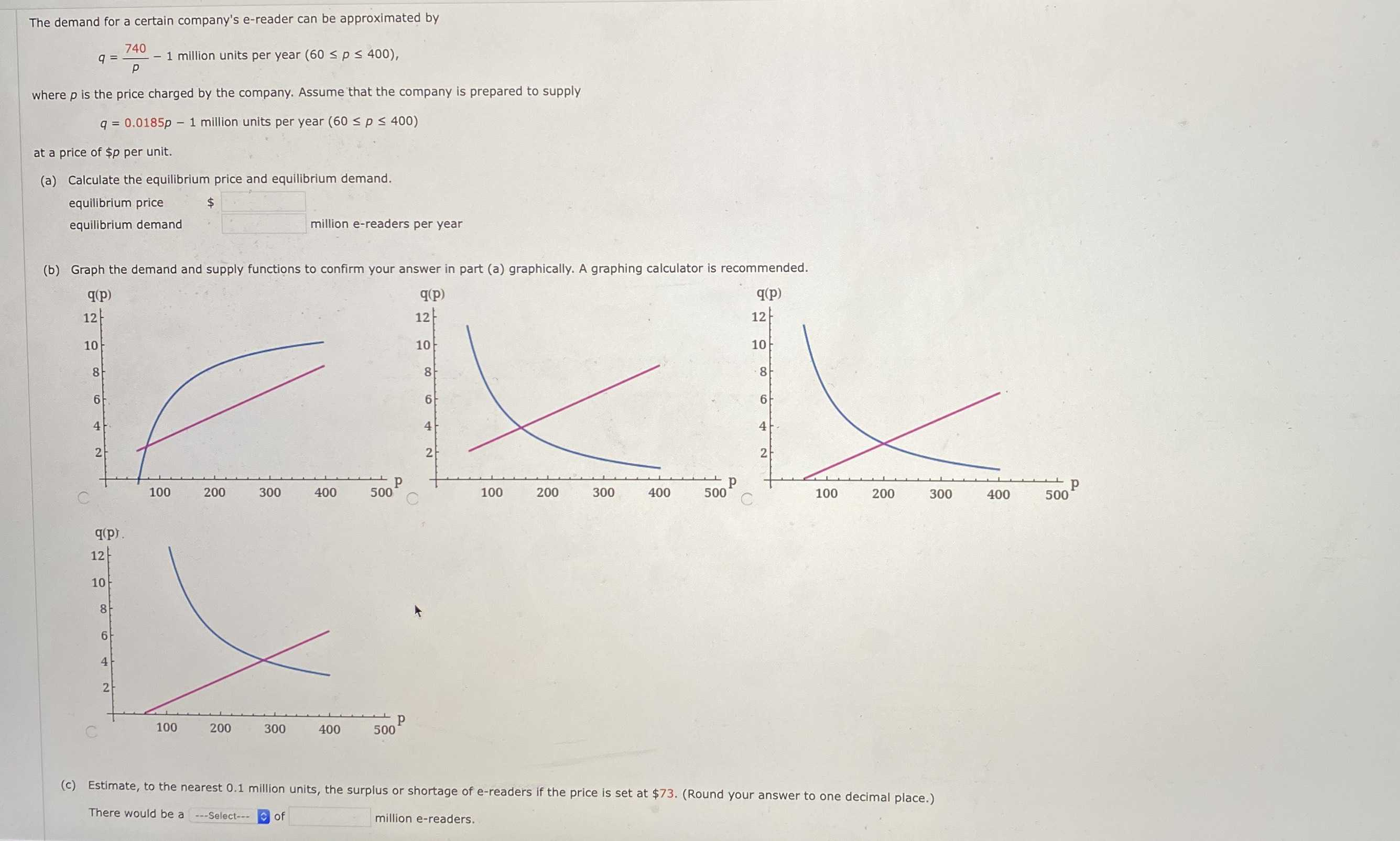### Still have math questions?

Algebra
QuestionThe demand for a certain company's e-reader can be approximated by

$$q = \frac { 740 } { p } - 1$$ million units per year $$( 60 \leq p \leq 400 )$$ ,

where $$p$$ is the price charged by the company. Assume that the company is prepared to supply

$$q = 0.0185 p - 1$$ million units per year $$( 60 \leq p \leq 400 )$$

at a price of $$\ p$$ per unit.

(a) Calculate the equilibrium price and equilibrium demand.

equilibrium price

equilibrium demand

(b) Graph the demand and supply functions to confirm your answer in part (a) graphically. A graphing calculator is recommended.

(a)

200

2.7

(b)

(c) shortage 8.8

Solution
View full explanation on CameraMath App.